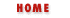# 5. Statements

Contents:

Select a section...

..then directly to it.

As we saw in the last chapter, expressions are JavaScript "phrases" that can be evaluated to yield a value. Operators within an expression may have "side effects," but in general, expressions don't "do" anything. To make something happen, you use a JavaScript statement, which is akin to a complete sentence or command.

A JavaScript program is simply a collection of statements. Statements usually end with a semicolon. In fact, if you place each statement on a line by itself, you may omit the semicolon. There are circumstances in which you are required to use the semicolon, however, so it is a good idea to get in the habit of using it everywhere.

The following sections describe the various statements in JavaScript and explain their syntax.

## 5.1 Expression Statements

The simplest kind of statements in JavaScript are expressions that have side effects. We've seen this sort of statement in the section on operators in Chapter 4, Expressions and Operators. One major category of these are assignment statements. For example:

```s = "Hello " + name;
i *= 3;
```

Related to assignment statements are the increment and decrement operators, ++ and --. These have the side effect of changing a variable value, just as if an assignment had been performed:

```counter++;
```

Function calls are another major category of expression statements. For example:

```alert("Welcome, " + name);
window.close();
```

These functions calls are expressions, but also produce an effect on the web browser, and so they are also statements. If a function does not have any side effects, then there is no sense in calling it, unless it is part of an assignment statement. So, for example, you wouldn't just compute a cosine and discard the result:

```Math.cos(x);
```

Instead, you'd compute the value and assign it to a variable for future use:

```cx = Math.cos(x);
```Miscellaneous Operators Book Index Compound Statements